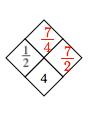### Home > CAAC > Chapter 1 > Lesson 1.1.4 > Problem1-36

1-36.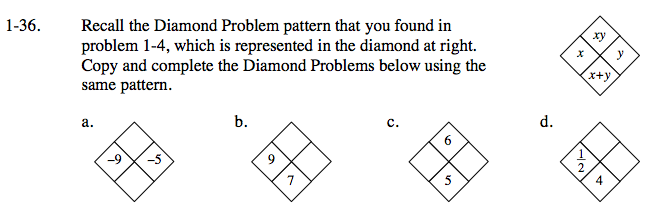Multiply the two given numbers together to get the top number. Add the given numbers together to get the bottom number.

The product of two negative numbers is positive. The sum of two negative numbers is negative.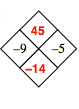What added to 9 equals 7?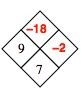What are the factors of 6? Which pair have a sum of 5?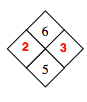Subtract one-half from 4 to find the right number.